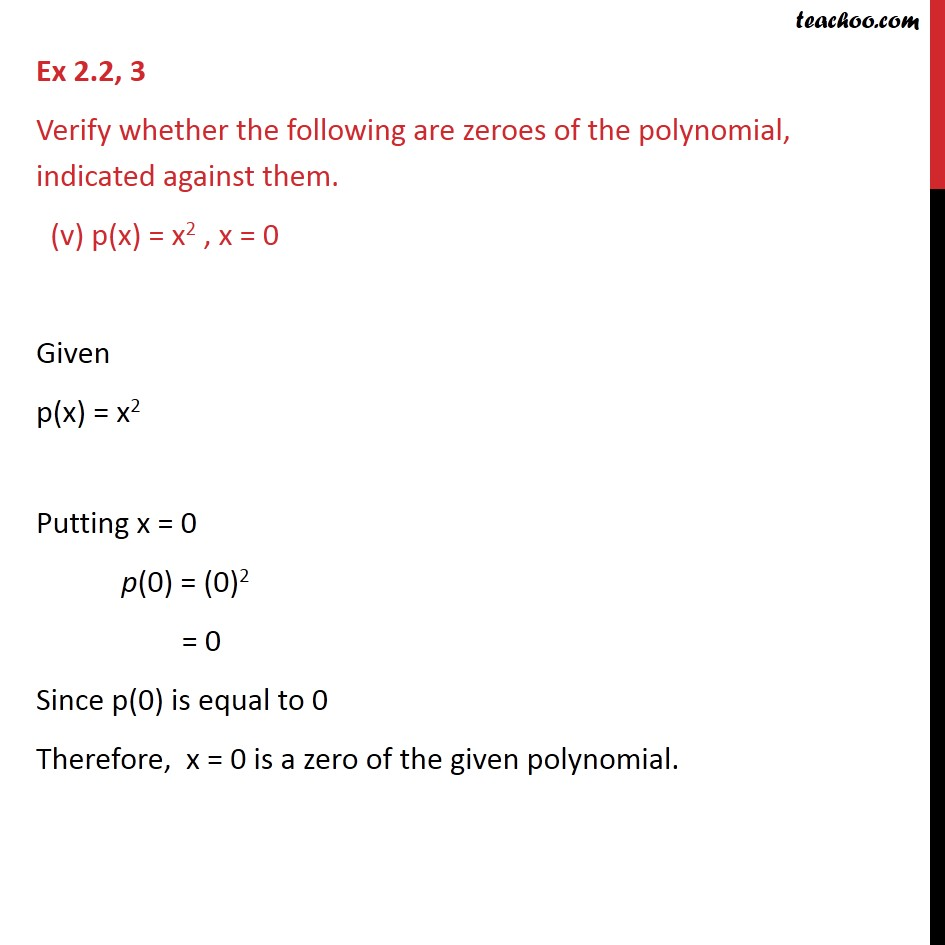Ex 2.2

Chapter 2 Class 9 Polynomials (Term 2)
Serial order wise### Transcript

Ex 2.2, 3 Verify whether the following are zeroes of the polynomial, indicated against them. (v) p(x) = x2 , x = 0 Given p(x) = x2 Putting x = 0 p(0) = (0)2 = 0 Since p(0) is equal to 0 Therefore, x = 0 is a zero of the given polynomial.